>>

 Math >> Arithmatic >> Mathematics >> Fraction
 New User Register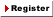Login Member: Email: Password:
 Site Search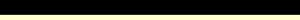Advance Search

Other animationsFractions - Fundamental concepts of FractionsAnimation product List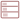Row formatGrid format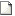List format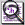Overview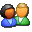(For age - group : Below 16 )

Fully animated ‘Fractions made easy’ takes the drudgery away from learning math and helps you to develop razor sharp concepts so that you can solve tough problems in a jiffy. Real life problems with detailed explanations and graphical depiction makes learning a pleasure.Product - Animation
 Size/Time Subscribe Preview / Trailer Size (KB) 9566 US\$    1.50 Rs. 108.00  Check price in your currency Subscription Days = 30 Watch a preview (opens in separate window) Time (hr:min:sec) 2:10:0 Add To CartPreview Unavailable

 This animation has audio.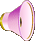Category : Math Fractions Type : Animation with sound Animation Type : Regular Total animation length: 2 Hour 10 minutes Watch combined preview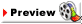The animation has the following broad sections: Understanding Fractions Comparing Fractions Converting Fractions Adding Fractions Subtracting Fractions Dividing Fractions Multiplying Fractions Reciprocal of a fraction Reducing Fractions Divisibility Rules Simplifying complex fractions Refer details section for the table of content.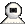Snapshots
 Watch combined preview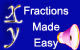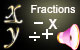Watch Fraction Made Easy or its other parts 1) Fundamental Concepts of Fractions 2) Kinds of Fractions 3) Mathematical Operations of Fractions shown above. Click on the above thumbnails to go to the fraction movie.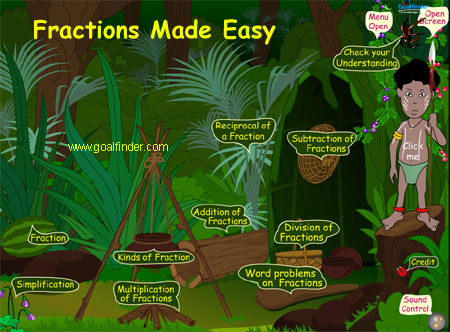Fractions find usage in every sphere of life; the animation of fraction has been prepared keeping in mind the confusions of students while solving math problems of fractions. This is an intuitive and interactive approach to fractions; there are short situational stories inside that explain the math concepts in a lucid manner inside a natural and real life environment.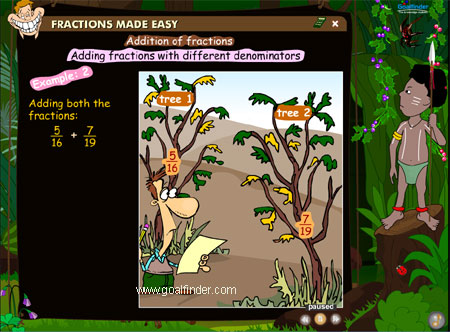This is like an animated math tutorial but with entertainment and stories to make learning fractions a fun. A systematic approach explains additions of fractions, subtraction of fractions, multiplication of fractions and division of fractions in a detailed style. An elaborate treatment of simplifying complex fractions clears the confusing math concept.Details of the animation/ movie /software

This animation contains all the three parts available as separate animations
1) Fundamental concepts of fractions
2) Kinds of fractions
3) Mathematical Operations of fractions

Following topics are covered in a detailed animated manner.

• What is fraction?
• What is a numerator in a fraction?
• What is a denominator in a fraction ?
• What is a bar?
• Proper fraction
• Improper fraction
• Mixed fraction
• How to change mixed to improper fraction
• Writing an improper fraction as a mixed number
• Negative fraction
• Simple fraction
• What is a whole number
• Complex fraction
• Decimal fraction
• Vulgar fraction
• Like fraction
• Equivalent fraction
• Unit fraction
• Greatest common factor
• Least common multiple
• Equivalent fractions
• Comparing fractions
• Converting and reducing fractions
• Lowest terms in fractions
• Mixed numbers
• Converting mixed numbers to improper fractions
• Converting improper fractions to mixed numbers
• Writing a fraction as a decimal
• Rounding a fraction to the nearest hundredth
• Adding fractions and subtracting fractions
• Adding fractions with the same denominator
• Adding fractions with different denominators
• Adding mixed fractions with the same denominator
• Subtract fractions with same denominators
• Subtract fractions with different denominators
• Adding and subtracting mixed numbers

• Multiplying fractions and whole numbers
• Multiplying fractions and fractions
• Multiplying mixed numbers
• Reciprocal of a fraction
• Dividing fractions
• Dividing mixed fractions
• Simplifying complex fractions
• Check your understanding section after chapters.Related Products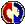Buyers Feedback: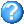Q & AGive your comments